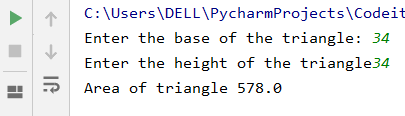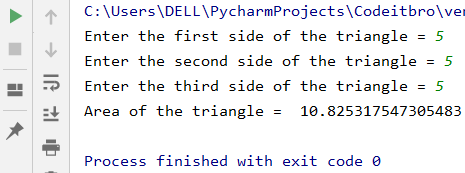# Python 3 Program to Calculate The Area of a Triangle

4478

In this Python tutorial, you will learn about a Python 3 program to calculate the area of a triangle. Apart from that, we will also explore:

• Python 3 program to find the area of a triangle with base and height
• Python 3 program to find the area of a triangle using all three sides
• Python 3 program to find the area of a triangle using function

To understand this program, you should have basic knowledge of the following Python programming languages:

• Syntax
• Variables and operators
• Python input and output

You can calculate the area of a triangle by numerous formulas depending on the metrics you know. For example, if you know the three sides of a triangle, then you can easily calculate are of a triangle using Heron’s formula.

We will see the Python code for each and every scenario to calculate the area of a triangle. To understand the theory, visit Mathisfun.

If you are looking for books to learn Python, check out this recommended list.

## Python 3 Program To Find The Area Of A Triangle With Base And Height

To calculate the area of a triangle whose base and height are known, you can use this formula = (Base x Height)/2.

Here’s the Python 3 program to calculate the area:

```base = input('Enter the base of the triangle:');  # Taking base input from user
base = float(base);  # Converting string input data to float type
height = input('Enter the height of the triangle');  # Taking height input user
height = float(height);  # Converting string input data to float type
Area = float((base * height) / 2);  # Calculateing the area of the triangle using (Base * Height)/2
print('Area of triangle', Area);  # Outputting the area of the triangle```

Output:## Python 3 Program To Calculate The Area Of A Triangle When All Three Sides Are Known

When you know all the three sides of a triangle, you can calculate the area of a triangle using Heron’s formula.

First, calculate the semi perimeter (S) by this formula – (Side 1 + Side 2 + Side 3)/2

After that, use this formula to calculate the area – √S*(S-Side1)*(S-Side2)*(S-Side3)

Here’s the Python program.

```import math
Side1 = float(input('Enter the first side of the triangle = ')) #First side of the triangle
Side2 = float(input('Enter the second side of the triangle = ')) #Second side of the triangle
Side3 = float(input('Enter the third side of the triangle = ')) #Third side of the triangle
S = float(((Side1+Side2+Side3)/2)) #Calculating semi perimeter
Area = float((S*(S-Side1)*(S-Side2)*(S-Side3))) #Solving Heron's expression within square root
Area = math.sqrt(Area) #Calculating the square root to find the area
print("Area of the triangle = ", Area) #Outputting the area of the triangle.```

Output:## Python 3 program to find the area of a triangle using function

• First, we will import the math Python library so that we can use the math.sqrt() function to calculate the square root of any number or expression.
• In this Python program, we will use Heron’s formula to calculate the area of a triangle.
• Heron’s formula = √S*(S-Side1)*(S-Side2)*(S-Side3), where S is the semi-perimeter of a triangle.
• We will define a Python function AreaTriangle(side1, side2, side3) to find the area of a triangle.

Code:

```import math
def TriangleArea(firstSide, secondSide, thirdSide):
SemiPerimeter = (firstSide + secondSide + thirdSide)/2
Area = math.sqrt((SemiPerimeter*(SemiPerimeter-firstSide)*(SemiPerimeter-secondSide)*(SemiPerimeter-thirdSide)))
print("Semi Perimeter of the triangle = %.2f" %SemiPerimeter);
print("Area of the triangle is %0.2f" %Area)
TriangleArea(3, 4, 5)```

Output:

Semi Perimeter of the triangle = 6.00
Area of a triangle is 6.00

## Programming Exercise:

Submit your programming exercise in the comments section, or you can also mail it to [email protected] Few lucky problem solvers will be featured on our Facebook pageCodeItBro – Programming Made Fun.

1. Write a Python program to calculate the area of a triangle when its two sides and the angle between them are known.

2. Make this AreaOfTriangles.py file into a Triangle Area Calculator, i.e., program will ask users how they would like to calculate the area of a triangle.